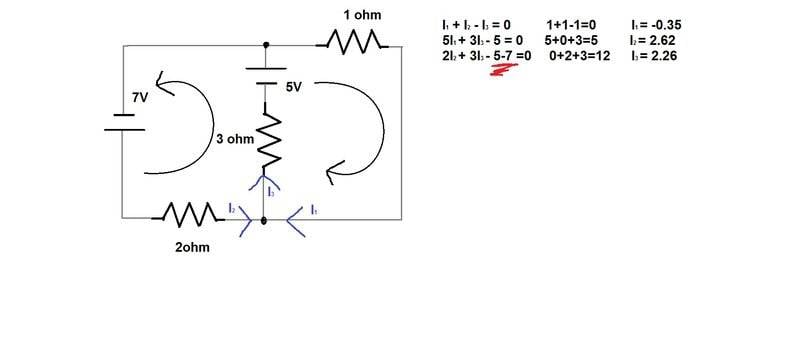# KVL/KCL with two sources - can a loop have both sources?## Homework Statement

In the circuit shown, find (a) the current in each resistors (b)The power dissipated in each resistor.

## Homework Equations

V=IR, P=VI, KVL and KCL

## The Attempt at a Solution

See attached.

I was going to post this in the main section as its really a question about whether you can have two voltage sources in your equation, but I read the sticky on the main page for general physics and it directed all coursework type questions here.

In the drawing I have a section underlined. When I set up my equations, I got two voltages in the equation. I wanted to know if that is valid? If I should come across another equation like this, is there a rule for knowing not to do this? The solution to this problem is not the same as what I derived. Please give me some direction with this, thanks!

## Answers and Replies

gneill
Mentor
Sure, a given loop can contain zero or more sources. For KVL their potential changes sum just as you've done.

•chopnhack
cnh1995
Homework Helper
Gold Member
In the drawing I have a section underlined. When I set up my equations, I got two voltages in the equation. I wanted to know if that is valid? If I should come across another equation like this, is there a rule for knowing not to do this? The solution to this problem is not the same as what I derived. Please give me some direction with this, thanks!
Your second equation is incorrect.
There should be i2 instead of i1.

•chopnhack
Sure, a given loop can contain zero or more sources. For KVL their potential changes sum just as you've done.
Thanks mate! BTW - if you haven't seen the finale.... jumping the shark abit... Still a fan though :-)

Your second equation is incorrect.
There should be i2 instead of i1.
Hi CNH, I think I see the error now. The 5I1 should really be just I1 times the 1 ohm resistor. That makes it all work.
Thanks gents!

SammyS
Staff Emeritus
Science Advisor
Homework Helper
Gold Member
Does it?

I1 flows through the 3 ohm resistance too. Plus, I2 also flows through the 3 ohm resistance. You should have all the three currents in that equation.
OP has 3 individual currents, each one for each branch of the circuit. He then uses a junction equation to relate them.

Last edited:
cnh1995
Homework Helper
Gold Member
It was almost 12 am when I posted it and I was kinda sleepy. I guess I have to sleep now before I post anything stupider.
OP has 3 individual currents, each one for each branch of the circuit. He then uses a junction equation to relate them.
Yes. I wasn't able to delete that because of network problems (I am traveling in a hilly area).
Fixed it now.

Last edited: